Next: 2.3.2 Mixture of probabilistic Up: 2.3 Mixture of local Previous: 2.3 Mixture of local

## 2.3.1 Gaussian mixture models

Gaussian mixture models assume that the patterns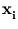origin from a probability density p(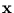) (Bishop, 1995). This density is a linear combination of Gaussian functions p(| j),

 p(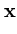| j) =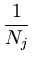exp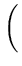-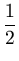(-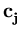)T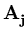(-)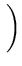. (2.16)

The normalization constant Nj is chosen such that the integral of p(| j) over IRd equals one (a necessary condition for a probability density). The negative exponent is a weighted squared distance (called Mahalanobis distance) betweenand the center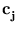; the corresponding weights are given by the symmetric matrix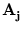. The boundary that has a Mahalanobis distance to the centerequal to one is a hyper-ellipsoid.

The density p() is a weighted sum of the local densities p(| j),

 p() =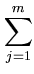P(j)p(| j) . (2.17)

To normalize p(), the weights P(j) must sum to one,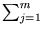P(j) = 1. Therefore, P(j) can be interpreted as the probability that patterns originate from the unit j. It is called prior probability.

The goal of the mixture model is to find the unknown parameters,, and the priors P(j) for each unit j such that the likelihood, L =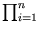p(), to obtain the distribution {} given the density p() is maximal (Bishop, 1995). To solve this optimization problem it is common to use a variant of the expectation maximization algorithm (Bishop, 1995). It consists of two steps with iterate until convergence is reached. In the expectation step, the soft-assignment P(j|) for all j and i is computed based on a given estimate of the parameters,, and P(j). P(j|) is called posterior probability. It is computed using Bayes' theorem (see appendix A.1),

 P(j|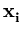) =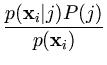. (2.18)

In the special case of uniform Gaussians that all have the same width and weight P(j), (2.18) is the same as the Gibbs distribution (2.14).

In the maximization step, the Gaussian's parameters,, and P(j) that maximize the likelihood given all P(j|) can be directly computed (Bishop, 1995). The result is that the centeris the weighted mean of the set {},=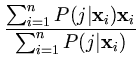, (2.19)

and the matrixis the inverse of the weighted covariance matrix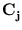,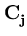=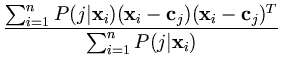. (2.20)

The inverse can be computed by extracting all eigenvectors of. Thus, the axes of the mentioned hyper-ellipsoid are the principal components of the local data distribution. The size of this hyper-ellipsoid is given by the eigenvalues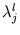from the PCA (the semi-axis length of unit j in the direction l equals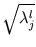). Finally, the result for the prior probabilities is

 P(j) =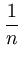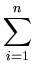P(j|) . (2.21)

It can be shown that alternating these expectation and maximization steps increases the likelihood L in each iteration step (Bishop, 1995). However, local maxima are not avoided. Further, single isolated data points (outliers) can make the algorithm unstable (Archambeau et al., 2003). If just one pattern is assigned to a unit (that is, the other patterns have almost zero P(j|)) the variance of the local Gaussian collapses to zero. As an improvement to the local minima problem, annealing schemes (as discussed for the vector quantization, section 2.2) were suggested (Albrecht et al., 2000; Meinicke and Ritter, 2001; Meinicke, 2000). Here, a global variance linked to the width of each Gaussian is gradually reduced during annealing.

The Gaussian mixture model as it is presented here has the disadvantage that all eigenvectors of the local covariance matrix need to be extracted. However, this problem can be overcome if probabilistic PCA is used instead of standard PCA.Next: 2.3.2 Mixture of probabilistic Up: 2.3 Mixture of local Previous: 2.3 Mixture of local
Heiko Hoffmann
2005-03-22